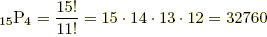index: click on a letter A B C D E F G H I J K L M N O P Q R S T U V W X Y Z A to Z index index: subject areas numbers & symbols sets, logic, proofs geometry algebra trigonometry advanced algebra & pre-calculus calculus advanced topics probability & statistics real world applications multimedia entrieswww.mathwords.com about mathwords website feedback

Permutation Formula

A formula for the number of possible permutations of k objects from a set of n. This is usually written nPk .

 Formula:Example: How many ways can 4 students from a group of 15 be lined up for a photograph? Answer: There are 15P4 possible permutations of 4 students from a group of 15.different lineups